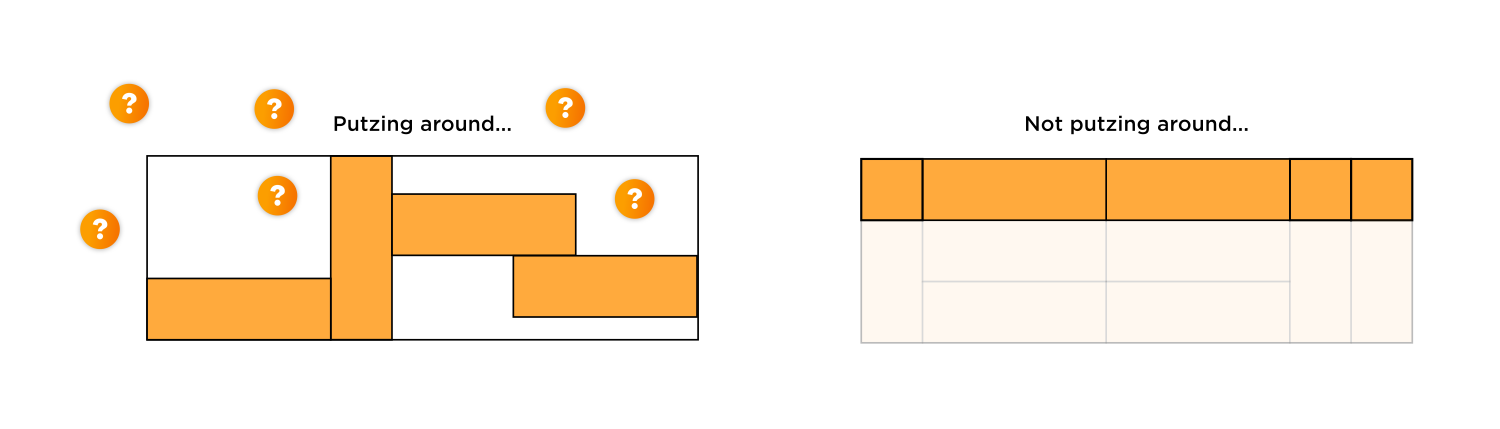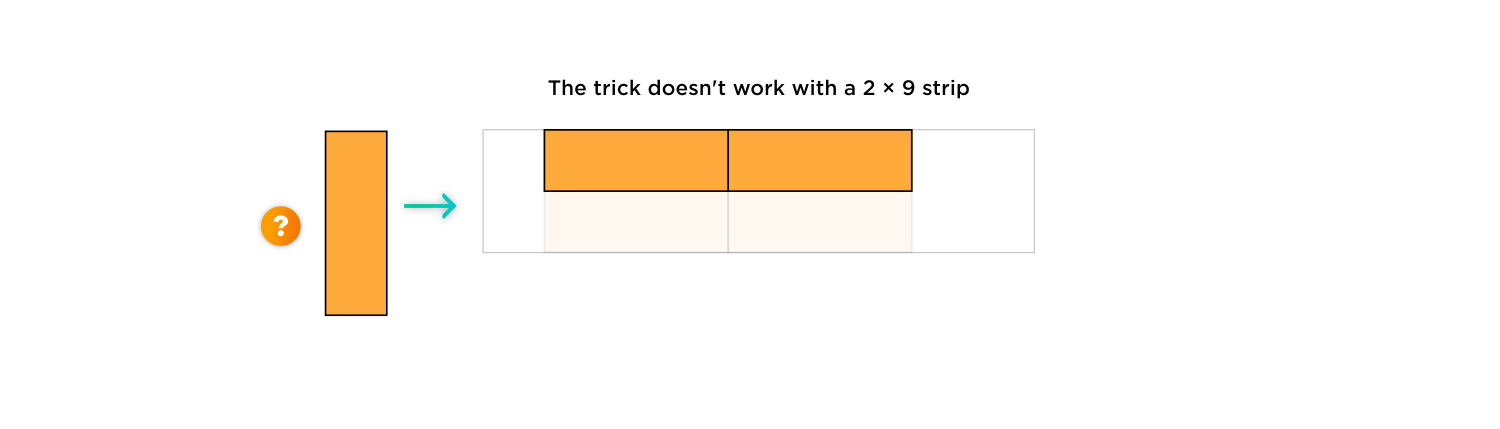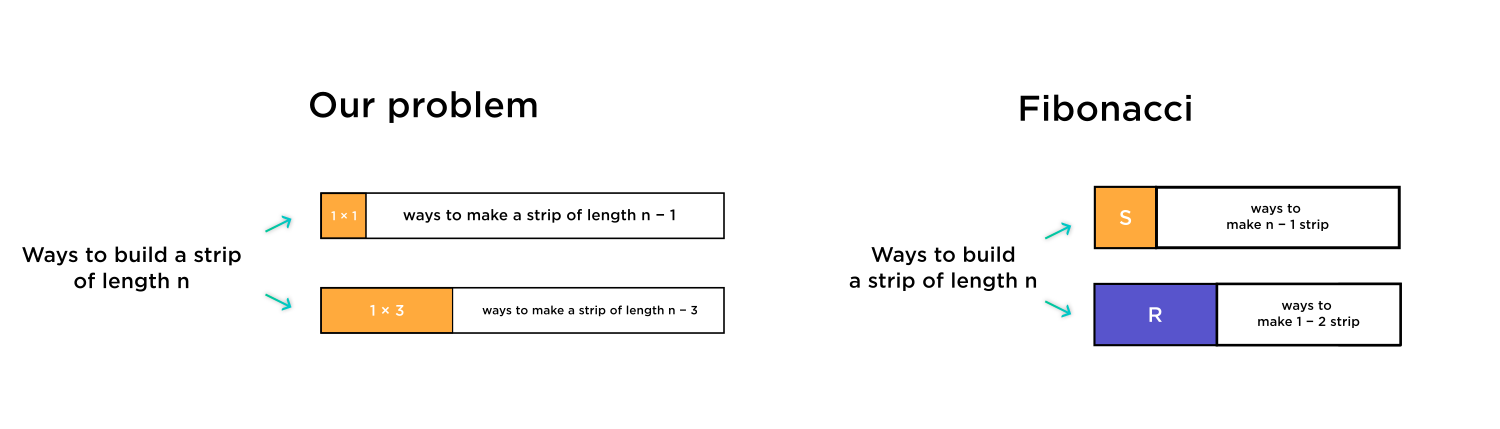# Does that recursion have anything to do with Fibonacci?

• Module 3 Week 3 Day 10 Your Turn Explanation Part 4

No idea what this explanation was about. Does that recursion have anything to do with Fibonacci?

• @The-Blade-Dancer This was a really cool problem!In Part 1, Prof. Loh showed us that tiling the 3-dimensional strip with width $$3$$ and length $$9$$ using just $$1 \times 3$$ rectangles is the same as tiling a 1-dimensional strip of length $$9.$$ That's really amazing! If I had never seen this problem before, I would probably have just putzed around for awhile trying to draw all the different ways to build the $$1 \times 3$$ strip.So Step 1 Of the Entire Problem is to actually forget about the whole $$3 \times 9$$ rectangle. Just look at the first row only! If we placed a vertical rectangle, think of this as instead a $$1 \times 1$$ square in the top row. If we placed a horizontal rectangle (with two identical rectangles under it), think of this instead as a single $$1 \times 3$$ rectangle in the top row.

( By the way, would this work with a $$2 \times 9$$ strip, if we were still tiling with $$1 \times 1$$ squares and $$1 \times 3$$ rectangles? It would not! You wouldn't be able to place a vertical $$1 \times 3$$ rectangle in such a skinny strip.)Okay, so we're going to banish the $$3 \times 9$$ rectangle now. It will no longer exist.We will simply think about how to tile a $$1 \times 9$$ strip with $$1 \times 1$$ squares and $$1 \times 3$$ rectangles.Step 2 of the Entire Problem is to think of a recursion for building such a strip. We could start with a $$1$$-length-shorter strip and add a $$1 \times 1$$ square, or start with a $$3$$-length-shorter strip and add a $$1 \times 3$$ rectangle.While the recursion formula isn't exactly the same as in the Fibonacci sequence, the reasoning behind how to get the formula is the same. The Fibonacci sequence applies to the case where we have $$1 \times 1$$ squares and $$1 \times 2$$ rectangles. Our problems is different; instead of $$1 \times 2$$ rectangles, we have $$1 \times 3$$ rectangles. If you understand the logic behind this, though, you can do any sort of recursion!The Fibonacci recursion formula was

$$a_n = a_{n-1} + a_{n-2}.$$

Our recursion formula is $$a_n = a_{n-1} + a_{n-3}.$$

Thus we can find the value of $$a_n$$ for small values of $$n,$$ like

$$a_1 = 1 \textcolor{blue}{ \text{ (the only way is a single square)}}$$
$$a_2 = 1 \textcolor{blue}{ \text{ (the only way is two squares)}}$$
$$a _3 = 2 \textcolor{blue}{ \text{ (either three squares or one rectangle)}}$$

From here on, we can use the recursion formula to find the successive values of $$a_n,$$ working our way up until we get to $$a_9,$$ which is what we want.

$$a_4 = a_3 + a_1 = 2 + 1 = 3$$
$$a_5 = a_4 + a_2 = 3 + 1 = 4$$
$$a_6 = a_5 + a_3 = 4 + 2 = 6$$
$$a_7 = a_6 + a_4 = 6 + 3 = 9$$
$$a_8 = a_7 + a_5 = 9 + 4 = 13$$
$$a_9 = a_8 + a_6 = 13 + 6 = \boxed{19}$$• @debbie wow :000